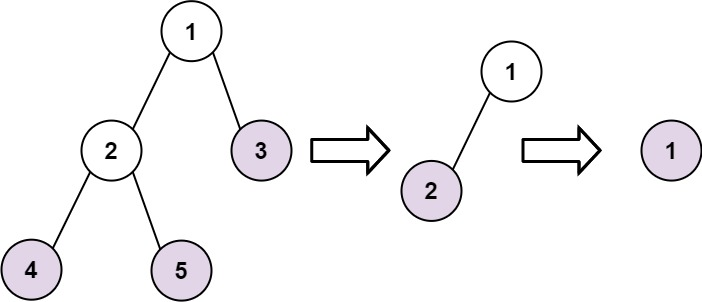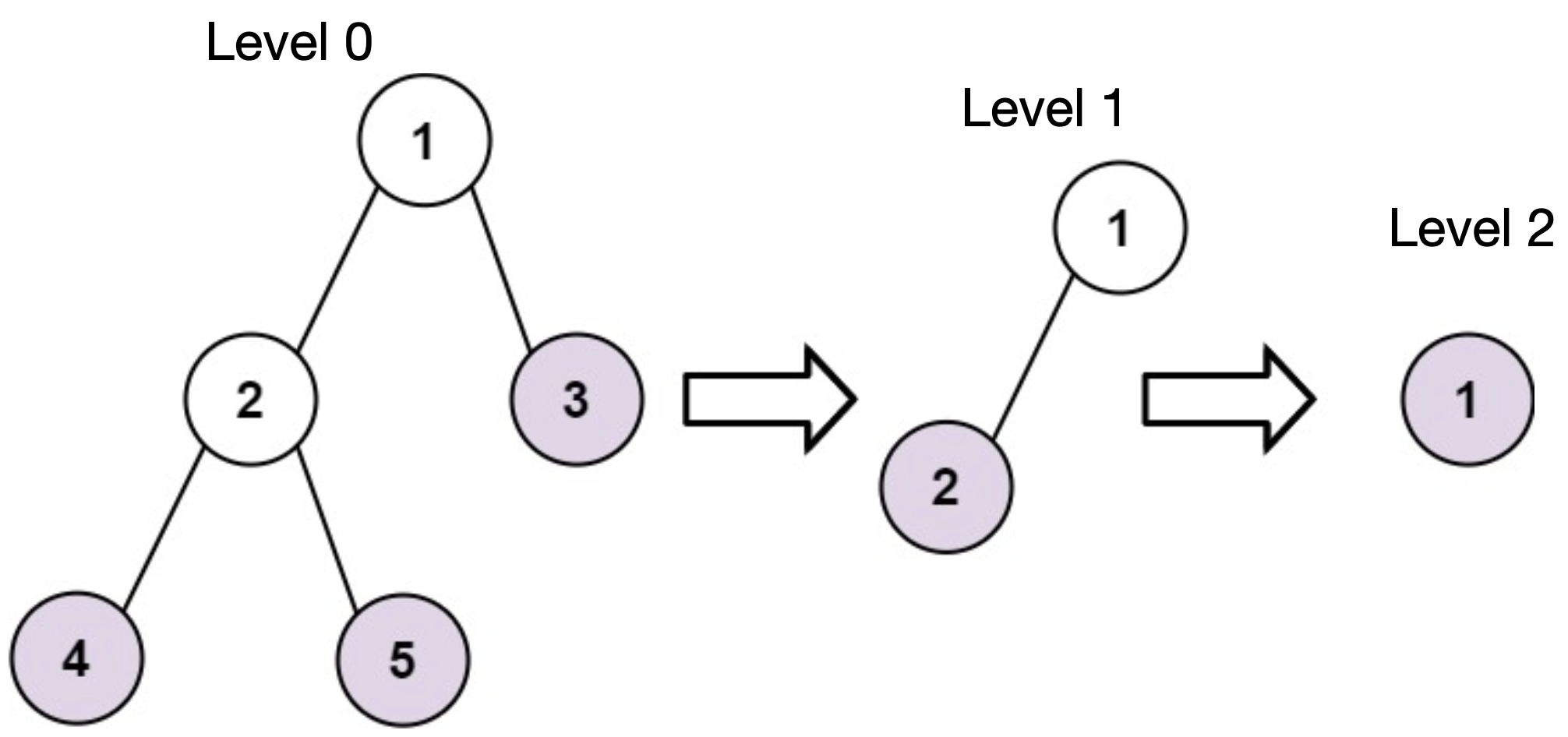# LeetCode 366. Find Leaves of Binary Tree Solution

Given the root of a binary tree, collect a tree's nodes as if you were doing this:

• Collect all the leaf nodes.
• Remove all the leaf nodes.
• Repeat until the tree is empty.

Example 1:Input: root = [1,2,3,4,5]
Output: [[4,5,3],,]
Explanation:
[[3,5,4],,] and [[3,4,5],,] are also considered correct answers since per each level it does not matter the order on which elements are returned.

Example 2:

Input: root =  Output: []

Constraints:

• The number of nodes in the tree is in the range [1, 100].
• -100 <= Node.val <= 100

## Brute Force

We can simply implement a solution that does what the problem asks one step at a time.

First, we will run a DFS to find all leaf nodes. Then, we'll remove them from the tree. We'll keep repeating that process until the tree is empty.

Let $N$ denote the number of nodes in the tree.

In the worst scenario (line graph), we will repeat this process $O(N)$ times and obtain a time complexity of $O(N^2)$.

However, a more efficient solution exists.

## Full Solution

Let's denote the level of a node $u$ as the step $u$ will be removed as a leaf node. For convenience, we will start counting steps from $0$.

### ExampleHere, nodes 3, 4, 5 have a level of $0$. Node $2$ has a level of $1$ and node $1$ has a level of $2$.

How do we find the level of a node?

One observation we can make is that for a node to be removed as a leaf in some step, all the children of that node have to be removed one step earlier. Obviously, if a node is a leaf node in the initial tree, it will be removed on step $0$.

If a node $u$ has one child $v$, $u$ will be removed one step after $v$ (i.e. level[u] = level[v] + 1).

However, if a node $u$ has two children $v$ and $w$, $u$ is removed one step after both $v$ and $w$ get removed. Thus, we obtain level[u] = max(level[v], level[w]) + 1.

For the algorithm, we will run a DFS and calculate the level of all the nodes in postorder with the method mentioned above. An article about postorder traversal can be found here. For this solution, we need to visit the children of a node before that node itself as the level of a node is calculated from the level of its children. Postorder traversal is suitable for our solution because it does exactly that.

### Time Complexity

Our algorithm is a DFS which runs in $O(N)$.

Time Complexity: $O(N)$

### Space Complexity

Since we return $O(N)$ integers, our space complexity is $O(N)$.

Space Complexity: $O(N)$

## C++ Solution

1/**
2 * Definition for a binary tree node.
3 * struct TreeNode {
4 *     int val;
5 *     TreeNode *left;
6 *     TreeNode *right;
7 *     TreeNode() : val(0), left(nullptr), right(nullptr) {}
8 *     TreeNode(int x) : val(x), left(nullptr), right(nullptr) {}
9 *     TreeNode(int x, TreeNode *left, TreeNode *right) : val(x), left(left),
10 * right(right)
11 * {}
12 * };
13 */
14class Solution {
15    vector<vector<int>> ans;  // ans[i] stores all nodes with a level of i
16   public:
17    int dfs(TreeNode* u) {    // dfs function returns the level of current node
18        if (u == nullptr) {
19            return -1;
20        }
21        int leftLevel = dfs(u->left);
22        int rightLevel = dfs(u->right);
23        int currentLevel =
24            max(leftLevel, rightLevel) + 1;  // calculate level of current node
25        while (ans.size() <=
26               currentLevel) {  // create more space in ans if necessary
27            ans.push_back({});
28        }
29        ans[currentLevel].push_back(u->val);
30        return currentLevel;
31    }
32    vector<vector<int>> findLeaves(TreeNode* root) {
33        dfs(root);
34        return ans;
35    }
36};
37
38

## Java Solution

1/**
2 * Definition for a binary tree node.
3 * public class TreeNode {
4 *     int val;
5 *     TreeNode left;
6 *     TreeNode right;
7 *     TreeNode() {}
8 *     TreeNode(int val) { this.val = val; }
9 *     TreeNode(int val, TreeNode left, TreeNode right) {
10 *         this.val = val;
11 *         this.left = left;
12 *         this.right = right;
13 *     }
14 * }
15 */
16class Solution {
17    List<List<Integer>> ans = new ArrayList<List<Integer>>();
18    // ans[i] stores all nodes with a level of i
19    public int dfs(TreeNode u) {
20        if (u == null) {
21            return -1;
22        }
23        int leftLevel = dfs(u.left);
24        int rightLevel = dfs(u.right);
25        int currentLevel = Math.max(leftLevel, rightLevel)
26            + 1; // calculate level of current node
27        while (ans.size()
28            <= currentLevel) { // create more space in ans if necessary
30        }
32        return currentLevel;
33    }
34    public List<List<Integer>> findLeaves(TreeNode root) {
35        dfs(root);
36        return ans;
37    }
38}

## Python Solution

1# Definition for a binary tree node.
2# class TreeNode:
3#     def __init__(self, val=0, left=None, right=None):
4#         self.val = val
5#         self.left = left
6#         self.right = right
7class Solution:
8    def findLeaves(self, root: Optional[TreeNode]) -> List[List[int]]:
9        ans = [[]]  # ans[i] stores all nodes with a level of i
10
11        def dfs(u):
12            if u == None:
13                return -1
14            leftLevel = dfs(u.left)
15            rightLevel = dfs(u.right)
16            currentLevel = (
17                max(leftLevel, rightLevel) + 1
18            )  # calculate level of current node
19            while len(ans) <= level:  # create more space in ans if necessary
20                ans.append([])
21            ans[level].append(u.val)
22            return level
23
24        dfs(root)
25        return ans
26